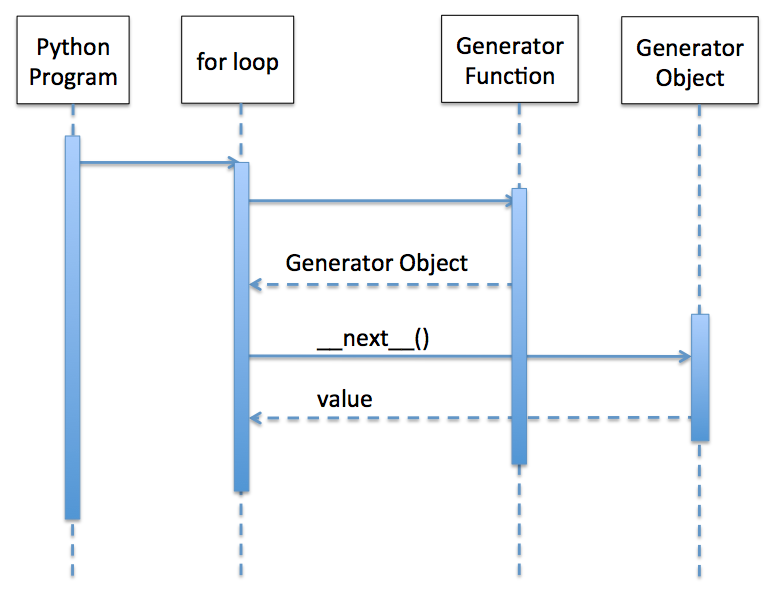# Generator Functions

## Regular Python Function:

A normal Python function passes back the computed value to the main flow of the program, through a return statement. Once the return statement is executed the values of the local variables of the function are lost and their associated resources are eventually garbage collected.

## Generator function in Python:

• In Python, a function with a yield statement is known as a generator function.
• The generator function is a form of coroutine.
• In contrast to the execution of a regular Python function with a return statement - a generator function with a yield statement returns a generator object, which supports the
• The iterator protocol is that when the __next__() function is called on the object, it returns a value from a sequence of values.
• The function with a yield statement after returning a generator object does not loose its local variables and resources. The variables are preserved. The execution of the generator function resumes upon a call to the __next__() method and returns the next value in the sequence.
• The above process continues till the function generates the last value of the sequence by marking it through an exception StopIteration along with the last value of the sequence. At this point, the generator function is exhaust.

## How a generator function works in Python:## Example 1:

 # A generator function def SimpleSequence():     for i in range(0, 5):             yield i;   # Get the generator object generatorObject = SimpleSequence(); try:     print(generatorObject);       # Print the first value in the sequence     print(generatorObject.__next__());       # Print the next values in the sequence     print(generatorObject.__next__());     print(generatorObject.__next__());     print(generatorObject.__next__());     print(generatorObject.__next__());     print(generatorObject.__next__());    except StopIteration:     print("End of Sequence");   # Same generator function executed using for loop    print("Printing through for loop:") for i in SimpleSequence():     print("i%d:%d"%(i,i))     # Python is powerful - another way of executing a generator function print("Another way of executing a generator function:") a,b,c,d,e = SimpleSequence(); print("a:%d b:%d c:%d d:%d d:%d"%(a,b,c,d,e));

## Output:

 0 1 2 3 4 End of Sequence Printing through for loop: i0:0 i1:1 i2:2 i3:3 i4:4 Another way of executing a generator function: a:0 b:1 c:2 d:3 d:4